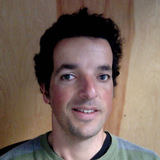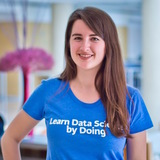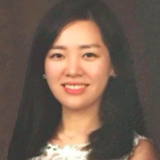Interactive Course

# Convolutional Neural Networks for Image Processing

Convolutional neural networks are deep learning algorithms that are particularly powerful for analysis of images.

• 4 hours
• 13 Videos
• 45 Exercises
• 8,553 Participants
• 3,650 XP

### Loved by learners at thousands of top companies:### Course Description

Deep learning methods use data to train neural network algorithms to do a variety of machine learning tasks, such as classification of different classes of objects. Convolutional neural networks are deep learning algorithms that are particularly powerful for analysis of images. This course will teach you how to construct, train and evaluate convolutional neural networks. You will also learn how to improve their ability to learn from data, and how to interpret the results of the training.

1. 1

#### Image Processing With Neural Networks

Free

Convolutional neural networks use the data that is represented in images to learn. In this chapter, we will probe data in images, and we will learn how to use Keras to train a neural network to classify objects that appear in images.

2. #### Going Deeper

Convolutional neural networks gain a lot of power when they are constructed with multiple layers (deep networks). In this chapter, you will learn how to stack multiple convolutional layers into a deep network. You will also learn how to keep track of the number of parameters, as the network grows, and how to control this number.

3. #### Using Convolutions

Convolutions are the fundamental building blocks of convolutional neural networks. In this chapter, you will be introducted to convolutions and learn how they operate on image data. You will also see how you incorporate convolutions into Keras neural networks.

4. #### Understanding and Improving Deep Convolutional Networks

There are many ways to improve training by neural networks. In this chapter, we will focus on our ability to track how well a network is doing, and explore approaches towards improving convolutional neural networks.

1. 1

#### Image Processing With Neural Networks

Free

Convolutional neural networks use the data that is represented in images to learn. In this chapter, we will probe data in images, and we will learn how to use Keras to train a neural network to classify objects that appear in images.

2. #### Using Convolutions

Convolutions are the fundamental building blocks of convolutional neural networks. In this chapter, you will be introducted to convolutions and learn how they operate on image data. You will also see how you incorporate convolutions into Keras neural networks.

3. #### Going Deeper

Convolutional neural networks gain a lot of power when they are constructed with multiple layers (deep networks). In this chapter, you will learn how to stack multiple convolutional layers into a deep network. You will also learn how to keep track of the number of parameters, as the network grows, and how to control this number.

4. #### Understanding and Improving Deep Convolutional Networks

There are many ways to improve training by neural networks. In this chapter, we will focus on our ability to track how well a network is doing, and explore approaches towards improving convolutional neural networks.

### What do other learners have to say?“I've used other sites, but DataCamp's been the one that I've stuck with.”

Devon Edwards Joseph

Lloyd's Banking Group“DataCamp is the top resource I recommend for learning data science.”

Louis Maiden

Harvard Business School“DataCamp is by far my favorite website to learn from.”

Ronald Bowers

Decision Science Analytics @ USAA##### Ariel Rokem

Senior Data Scientist, University of Washington

Ariel Rokem is a Data Scientist at the University of Washington eScience Institute. He received a PhD in neuroscience from UC Berkeley, and postdoctoral training in computational neuroimaging at Stanford. In his work, he develops data science algorithms and tools, and applies them to analysis of neural data. He is also a contributor to multiple open-source software projects in the scientific Python ecosystem.

See More
##### Collaborators
•Lore Dirick

•Eunkyung Park

•Sumedh Panchadhar